# Performance of the Optimal Nonlinear PID Controller for Position Control of Antenna Azimuth Position System

Performance of the Optimal Nonlinear PID Controller for Position Control of Antenna Azimuth Position System
Control and Systems Engineering Department, University of Technology- Iraq, Baghdad 10066, Iraq

Biomedical Engineering Department, University of Technology- Iraq, Baghdad 10066, Iraq

Corresponding Author Email:
luay.t.rasheed@uotechnology.edu.iq
Page:
366-375
|
DOI:
https://doi.org/10.18280/mmep.100143
9 October 2022
|
Accepted:
25 January 2022
|
Published:
28 February 2023
| Citation

OPEN ACCESS

Abstract:

This study presents the improvement in the performance of the Proportional-integral-derivative (PID) controller for position control of antenna azimuth position system subjected to external disturbance. The design of the PID controller is developed by adding the arc tan function of error instead of the direct error in an integral part of the PID controller, yielding a Nonlinear PID controller (NPID). A Particle Swarm Optimization (PSO) is used in this study to tune the parameters of the PID and NPID controllers using the Root Mean Square Error (RMSE) cost function. The simulations have been accomplished under the MATLAB/Simulink environment. The simulation results show that a PSO-based NPID controller provides superior steady and transient state performance compared to a PSO-based PID controller. In addition, the effectiveness of the proposed controller is verified via numerical simulation compared to the performance of other controllers with and without external disturbance.

Keywords:

antenna azimuth position control system, PSO algorithm, PID controller, nonlinear PID controller

1. Introduction

The modern world is currently reliant on control systems. Control system concepts are used in numerous applications in our environment. Robotics, automatic lifts, rocket fire, space shuttle lifts off to earth, splashing cooling water, car hydraulic pistons, and many other real-world applications are examples of such applications. Generally, the traditional PID controller is regarded as the industry's most advanced type of feedback control. This is primarily due to its simplicity, transparency, reliability, and high efficiency. However, classical controllers have some drawbacks, such as a decrease in performance as system order increases, sensitivity to changes in system parameters, and poor performance with nonlinear systems. Therefore, different control techniques can be used to overcome these problems [1, 2].

A position control system converts an input position command to the desired output position response. Robot arms, antennas, and computer disk drives contain many applications for the position control system. The antenna azimuth system is a servo-controlled mechanism comprised of gears and feedback potentiometers . Figure 1 depicts the physical layout model of the antenna azimuth control system . Many researchers have offered various control techniques to address the position control problem for antenna azimuth systems. The following literature review examines the most relevant works addressing the problem of position control in antenna azimuth systems.

Uthman and Sudin  have proposed a state feedback controller and PID controller to enhance the overall position control of antenna azimuth position systems. The gains of the PID controller were tuned using the Ziegler-Nichols method. Using the state-space representation, the state feedback controller was derived, and the pole placement method was utilized to guarantee that the controlled system met the requirements for the transient response. Mahmood et al.  have proposed a genetic algorithm-based FOPID controller for the position control of the antenna azimuth system. The genetic algorithm is used with different types of cost functions, including Integral Time Square Error (ITSE), Integral Square Error (ISE), and Mean Square Error (MSE).

Kumar et al.  have presented the design of a lead compensator (LC), fractional order lead compensator (FOLC), and proportional and integral (PI) controller for the position control of the antenna azimuth system. The fractional order calculus is crucial for the design of robust control. The parameters of the PI controller were tuned using the Ziegler-Nichols method, and the LC was designed based on the desired phase margin. Okumus et al.  have used the design of a traditional PID controller, fuzzy logic controller (FLC), and a self-tuning fuzzy logic controller (STFLC) for the position control of the antenna azimuth system. Different types of fuzzy membership functions and different numbers of fuzzy rules are examined in order to obtain the best system response. The PID controller variables are obtained using the try and error method.

Aloo et al.  have used the design of PID controller, Linear Quadratic Regulator (LQR), and hybrid PID-LQR controller for addressing the antenna azimuth position control problem. The PID variables are determined using the Ziegler Nichols tuning method. Fandakli and Okumuş  have presented the design of FLC, PID controller, and sliding mode controller (SMC) for the antenna position control system. The PID variables are obtained using the Ziegler Nichols tuning method.

## 1.png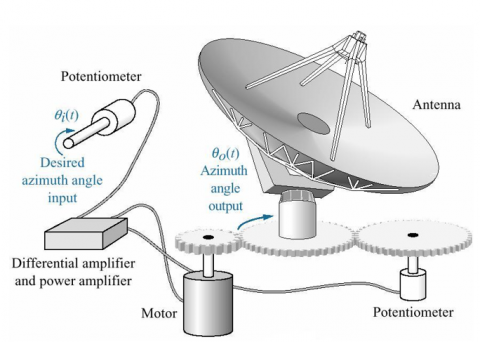Figure 1. The physical layout model of the system 

Singh and Pal  have applied a Model Reference Adaptive Controller (MRAC) and Self-tuning Controller (STC) for the antenna position control system. These controllers are capable of adapting to changing environmental conditions and minimizing the antenna's deviation from its desired position. Eze et al.  have used a positioning control strategy for the antenna position control system using PID tuned compensator (PIDTC). The PIDTC is capable of providing robust performance in the presence of external disturbance.

The motivation of this work is that the problems facing the antenna azimuth positioning control have been the subject of recent continuous and rigorous research for researchers. Therefore, the need for a high-performance controller for the antenna azimuth positioning control is a challenging control problem. To enhance the dynamic response of the closed-loop system, a nonlinear PID (NPID) controller is considered in this work. The selection of the PID parameters using a trial-and-error method does not give an optimal solution. Therefore, different optimization algorithms can be utilized to tune these parameters, such as Spider Monkey Optimization (SMO), Firefly algorithm (FA), Grey Wolf Optimization (GWO), Sine Cosine Algorithm (SCA), Genetic Algorithm (GA), Whale Optimization Algorithm (WOA), etc. In this work, the PSO algorithm is utilized to determine the optimum gains of the PID and NPID controllers because of its fast convergence, the efficiency of computation, and its capability to reach global solutions [9, 10].

The main contributions of this study are highlighted by the following points:

•     Improve the performance of the PID controller for the position control of the antenna azimuth system. This improvement has been made by adding the arc tan function of error instead of the direct error in an integral part of the PID controller to form the NPID controller.

•     Design of PSO algorithm to optimally tune the parameters of the PID and NPID controllers in order to enhance the dynamic performance of the controlled system.

This paper is structured into six sections: Section 2 describes the derivation of the systems transfer function. Section 3 discusses the proposed NPID controller design. Section 4 discusses in detail the PSO technique to find the optimal gains of the PID and NPID controllers. In section 5 the simulation results of control techniques are discussed. Finally, the conclusions are highlighted in section 6.

2. Modeling of the System

This system is divided into five major subsystems. The power amplifier serves as the motors drive circuit, and potentiometers are utilized to convert to voltages the azimuth angles (in degrees). The motor and the load are combined into a single system, and its formula includes all of the required parameters. Finally, the gearbox increases the applied torque while decreasing the speed and vice-versa by changing the gear ratio . Figure 2 depicts the schematic diagram of the physical subsystems with their parameters and internal connections .

The derivation of the antenna’s DC motor transfer function is taken from the research . Therefore, the motor and load transfer function can be expressed in Eq. (5) using Eqns. (1)-(4).

$K_m=\frac{K_t}{J R_a}$    (1)

$a_m=\frac{D_m R_a+K_b K_t}{J R_a}$    (2)

$J=J_a+J_L\left(K_g\right)^2$    (3)

$D_m=D_a+D_L\left(K_g\right)^2$    (4)

$\frac{\emptyset_m(s)}{E_a(s)}=\frac{K_m}{s\left(s+a_m\right)}$    (5)

The power amplifier block’s transfer function can be defined as follows :

$\frac{E_a(s)}{V_p(s)}=\frac{K_1}{s+a}$    (6)

## 2.pngFigure 2. The physical layout model of the system 

## 3.png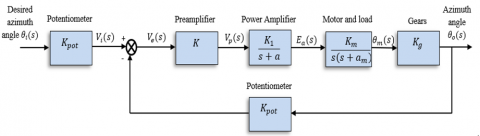Figure 3. The physical layout model of the system 

Table 1. The antenna schematic diagram parameters 

 Parameter and Symbol Value and Unit Power Amplifier Pole (a) 100 Motor and Load Pole (am) 1.71 Dampening Constant of The Motor (Da) 0.01(Nms/rad) Dampening Constant of The Load (DL) 1(Nms/rad) Equivalent viscous friction coefficient (Dm) 0.02(Nms/rad) Motor Inertial Constant (Ja) 0.02(Kgm2) Load Inertial Constant (JL) 1(Kgm2) Equivalent moment of inertia (J) 0.03(Kgm2) Power Amplifier Gain (K1) 100 Preamplifier Gain (K) --- Back emf Constant (Kb) 0.5(V/rad/s) Gear Ratio (Kg) 0.1 Motor and Load Gain (Km) 2.083 Motor Armature Resistance (Ra) 8(Ω) Potentiometer Gain (Kpot) 0.318 Motor Torque Constant (Kt) 0.5(Nm/A) The number of teeth in gears N1 and N2, respectively 25, 250 The armature voltage of the DC-servo motor (Ea) ±48(V)

The gear ratio in Eq. (7) increases the motor’s speed while decreasing its torque .

$K_g=\frac{N_1}{N_2}$    (7)

Multiplying the power amplifier block, motor and load block, and gear ratio block and using the values of their parameters in Table 1 gives the open-loop transfer function of the systems. Thus, the open-loop transfer function between the angular position and the input voltage is defined in Eq. (8) .

$\frac{\emptyset_o(s)}{V_p(s)}=\frac{20.83}{s^3+101.71 s^2+171 s}$    (8)

The value of the preamplifier gain "K" can be obtained using the Routh-Herwitz stability criterion that achieves the system’s stability. Based on this method, if the preamplifier gain’s value "K" is within the range of 0-262.3, the response of the system could be considered stable . Depending on the research , the optimal value of "K" is 100 because it yields the best response.

The comprehensive block diagram for the physical layout model of the system is illustrated in Figure 3 . Table 1 illustrates the antenna schematic diagram’s parameters .

3. Controllers Design

The PID controllers are widely used in industrial applications to improve both transient and steady-state behaviors of the controlled system due to their simple control structure, high accuracy, and high robustness. There are three parameters in the structure of the PID controller, namely the proportional gain Kp, integral gain Ki, and the differential gain Kd. These three gains have a significant impact on the control systems’ performance. Therefore, their values should be chosen carefully [13, 14]. The time domain formulation of a PID controller is as follows :

$u(t)=K p e(t)+K i \int e(t) d t+K d \frac{d e(t)}{d t}$    (9)

where, Kp is the proportional parameter, Ki is the integral parameter, Kd is the derivative parameter, and e is the error signal.

The NPID controller structure is given by replacing the integral for the error function in Eq. (9) with the integral for the arc tan function of the error. When the disturbance affecting the system dynamics is constant, the traditional PID controller is sufficient because the control signal forces the state to track the desired reference. When the disturbance term is not constant, the linear PID can only attenuate the effect of the disturbance with a linear integral control term. The ability to attenuate disturbances in linear or nonlinear PID structures is primarily determined by the integral term, as its effects are integrated and increased with time to reject the disturbance . Accordingly, the proposed NPID control law using the arc tan function in the integral term is as follows :

$u(t)=K p e(t)+K i \int \tan ^{-1}(\gamma e(t)) d t+K d \frac{d e(t)}{d t}$    (10)

where, γ is a design parameter.

Figure 4 depicts the system’s block diagram that controls the antenna azimuth’s angular position.

4. Particle Swarm Optimization

Kennedy and Eberhart introduced PSO in 1995 as a swarm intelligence method for addressing optimization problems and finding local and global solutions. The fundamental PSO algorithm relies on three steps (generating particles’ positions and velocities, updating the particles’ velocity, and updating the particles’ position). This algorithm is inspired by the behaviour of some animals, such as fish and birds. The following equations govern the velocity and position updates of each particle [15, 16]:

$V_i^{k+1}=w_1 V_i^k+c_1 r_1\left(p_{\text {best }_i}^k-X_i^k\right)+c_2 r_2\left(g_{\text {best }}^k-X_i^k\right)$    (11)

$X_i^{k+1}=X_i^k+V_i^{k+1}$    (12)

where, i=1,2, …, Np, Np is the population size, k=1,2,…, kmax, kmax is the maximum iterations number, $V_i^k$ is the velocity of the ith particle at kth iteration, w1 is the inertia weight, c1 is the self-confidence weight, c2 is the swarm confidence weight, r1 and r2 are random numbers between [0,1], pbest is the particle's best position found at kth iteration, $g_{b e s t}^k$ is the best position found by all particles at kth iteration, and $X_i^k$ is the location of the ith particle at kth iteration.

During the search for the minimum, the Root Mean Square Error (RMSE) is selected as a cost function for evaluating each particle, which is defined as follows :

$R M S E=\sqrt{\frac{1}{n} \sum_{i=1}^n(R-Z)^2}$    (13)

where, R is the reference signal, Z is the actual signal, and n is the number of acquired samples.

## 4.png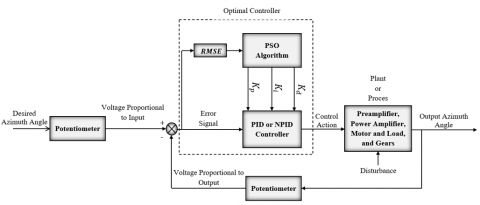Figure 4. The controlled system block diagram

The following pseudocode represents the PSO technique to tune the gains of the PID and NPID controllers:

Step 1: Initialization.

1. Initialize the PSO parameters, such as the population size (Np), the inertial weight coefficient (w1), learning factors (c1 and c2), and the maximum iterations number (kmax).
2. for every particle i=1,…,Np, do

Initialize the position and velocity of particles randomly: Xi(0), and Vi(0).

1. Evaluate the cost function (RMSE) for all particles using Eq. (13).
2. Set pbest to its initial position: $p_{\text {best }_i}=$ Xi(0).
3. Set gbest to the position of the particle that has a minimum RMSE value among all particles.

end for

Step 2: Repeat until the maximum iteration number is reached.

while (k<kmax) do

for each particle i=1,…,Np, do

Update the particles velocity using Eq. (11).

Update the particles position using Eq. (12).

Evaluate the RMSEi function using Eq. (13).

if $R M S E\left(X_i^{k+1}\right)<\operatorname{RMSE}\left(X_i^k\right)$.

$p_{\text {best }_i}=X_i^{k+1}$.

end if

if $\min \left(\mathrm{R} M S E\left(X^{k+1}\right)<\min \left(\mathrm{R} M S E\left(X^k\right)\right.\right.$

$g_{\text {best }}=X_{\min (R M S E)}^{k+1}$  (the position of the particle with a minimum $R M S E$  value among all particles).

end if

end for

k=k+1

end while

Step 3: Output gbest that contains the best-found solution.

5. Simulation Results and Discussion

In this section, the simulation results for the PID and NPID controllers based on the PSO technique are presented for the tracking control of the antenna azimuth model. The effectiveness of both optimal controllers is examined using computer simulation within the MATLAB program. The antenna azimuth systems parameter values are illustrated in Table 1.

The states of the antenna azimuth system are x1, x2, and x3 and represent the angular position (θ) in (rad), the angular velocity ( $\dot{\theta}$ ) in (rad/sec), and the armature current (ia) in (amp), respectively. The simulation initial values of the states for both optimal controllers are selected as illustrated below:

$\left[\theta(0), \dot{\theta}(0), i_a(0)\right]^T=[0,0,0]^T$

The open-loop output position step response of the antenna azimuth model is depicted in Figure 5. This Figure demonstrates that the response of the system is unstable.

The PSO algorithm is used to determine the optimum gains of the controllers. These design parameters allow the controllers to generate the optimal voltage control signal that minimizes the position tracking error with the minimum number of fitness evaluations. The setting parameters of the PSO technique are defined in Table 2.

The simulation results of controlled system with the desired output position of 1 radian and no external disturbance are depicted in Figures 6-10. The output position responses of the antenna azimuth system using both optimal controllers exhibit no overshoot in the transient state, and the steady-state error is zero, as depicted in Figure 6. In addition, the system controlled by a PSO-based NPID controller has less settling time than that controlled by a PSO-based PID controller.

The optimal parameters of the controllers have illustrated in Table 3. The dynamic behaviour of the controlled system, including settling time, overshoots, and steady-state error, are set in Table 4. Figure 6 and Table 4 reveal that the dynamic behaviour of the PSO-based NPID controller is superior to that of the PSO-based PID controller. Moreover, Table 4 demonstrates that the PSO-based NPID controller outperforms other controllers .

Table 2. The design parameters of the PSO algorithm

 Description and Symbol Value Population Size (Np) 25 Maximum number of iterations (kmax) 100 Cognitive Parameter (c1) 1.49618 Social Parameter (c2) 1.49618 Inertia Factor (w1) 0.7298 The Problem dimension 3 for PID Controller 4 For NPID Controller

Table 3. Optimal values of the controllers gains based on PSO

 Parameter PSO-based PID controller PSO-based NPID controller Kp 6.015 28.87 Ki 0.0001 0.0001 Kd 1.502 6.612 γ --- 10.05

Figure 7 shows the responses of voltage control actions of both optimal controllers. The voltage control actions are smooth without oscillation response, and no sharp spikes behaviour occurred. Moreover, the voltage control actions do not exceed the acceptable range of (±48) volts depending on the supply voltage of the DC servomotor of the antenna azimuth system.

Table 4. Dynamic performances of the controlled system using the optimal controller

 Parameter PSO-based PID controller PSO-based NPID controller state feedback controller  Settling Time (Ts) 1.7 sec 1.2 sec 3 sec Maximum Peak Overshoot (Mp) 0 0 5% Error Steady-State (Es.s) 0 0 0 RMSE 0.05233 0.05055 -

## 5.pngFigure 5. The step response of the open-loop system

## 6.png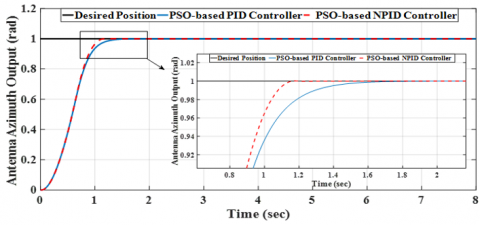Figure 6. The dynamic responses based on optimal controllers with time

## 7.png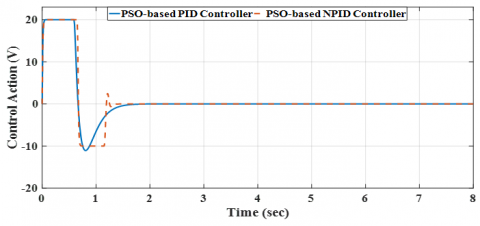Figure 7. The voltage control signals of the optimal controllers with time

## 8.png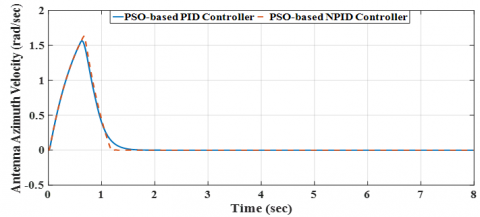Figure 8. The angular velocities of the controlled system with time

## 9.png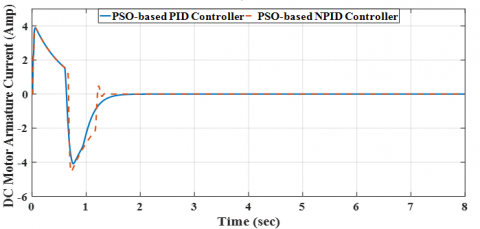Figure 9. The armature currents of the antenna azimuth system's DC servomotor with time

## 10.pngFigure 10. The behaviours of the (RMSE) cost function with iteration for optimal PID and NPID controllers

## 11.png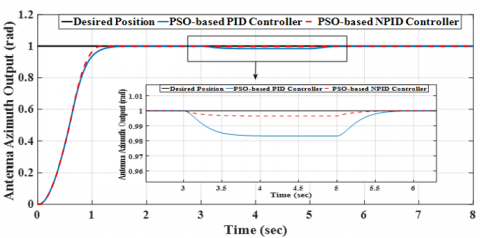Figure 11. The dynamic responses based on optimal controllers with time under disturbance

## 12.png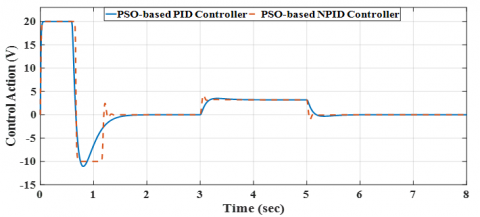Figure 12. The voltage control signals of the optimal controllers with time under disturbance

## 13.png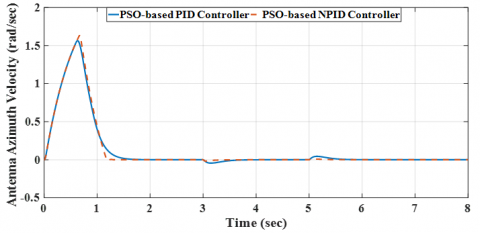Figure 13. The angular velocities of the controlled system with time under disturbance

## 14.pngFigure 14. The armature currents of the DC servomotor of the antenna azimuth system with time under disturbance

The angular velocity (x2) state of the antenna system and the armature current (x3) state of the DC-servo motor of the antenna system are depicted in Figures 8 and 9, respectively. These figures demonstrate that the angular velocity of the system controlled by a PSO-based NPID controller is faster than that of the system controlled by a PSO-based PID controller. In addition, the system controlled by a PSO-based NPID controller consumes less current than that of the system controlled by a PSO-based PID controller.

Figure 10 depicts the improved cost function (RMSE) of the controlled system using both optimal controllers after 100 iterations.

An external torque disturbance (Td) equal to (0.2 N.m) is added at the moment (3-5 sec) to investigate the robustness performance of the controlled system. The response of the systems output position using a PSO-based NPID controller has a smaller overshoot than that of a PSO-based PID controller during disturbance addition, and the error equals zero value at the steady state response, as depicted in Figure 11. The control action responses of both optimal controllers are shown in Figure 12.  This figure indicates that the control action responses are able to track the error position signal of the antenna azimuth system following the desired position and reduce the torque external disturbance effect. Finally, the states of the angular velocity (x2) and the armature current (x3) of the antenna azimuth system are depicted in Figures 13 and 14, respectively. These figures show that there is a small oscillation in their responses while adding the external disturbance.

6. Conclusions

This study has conducted a comparison study between two optimized controllers, PSO-based PID and PSO-NPID controllers, for controlling the angular position of antenna in azimuth channel. The robustness of both controllers has been assessed in the presence of exerted load. Numerical simulation based on MATLAB has been used for evaluating the effectiveness of both controllers, in addition, the comparison study has been extended to include other state-feedback controller as a third competitor. The simulated results showed that the tracking performance of PSO-based NPID controller outperforms both PSO-based PID controller and state feedback controller in terms of transient characteristics. Moreover, when the system is subjected to an external torque disturbance, the PSO-based NPID controller has shown better robustness characteristics as compared to its counterparts.

In order to extend this study for future work, one may suggest other modern optimization techniques like Social Spider Optimization (SSO), butterfly optimization algorithm (BOA), and Grey-Wolf Optimization (GWO) [17-19]. A comparison study can be conducted between the results obtained from the proposed PSO algorithm and those obtained from the suggested optimization methods. In addition, different control techniques can be used and designed to control the angular position of the radar and a comparison study can be conducted between these suggested controllers and the proposed controller [20-31].

Nomenclature
 a dimensionless power amplifier pole am dimensionless motor and load pole c1 dimensionless self-confidence weight c2 dimensionless swarm confidence weight Da dampening constant of the motor, Nms/rad DL dampening constant of the load, Nms/rad Dm equivalent viscous friction coefficient, Nms/rad Ea the armature voltage of the DC-servo motor, V e error signal, V Ja motor inertial constant, Kgm2 JL load inertial constant, Kgm2 J equivalent moment of inertia, Kgm2 K1 dimensionless power amplifier gain K dimensionless preamplifier gain Kb back emf constant, V/rad/s Kg dimensionless gear ratio Km dimensionless motor and load gain Kpot dimensionless potentiometer gain Kt motor torque constant, Nm/A Kp dimensionless proportional gain Ki dimensionless integral gain Kd dimensionless derivative gain N1 and N2 dimensionless number of teeth in gears Ra motor armature resistance, Ω r1 dimensionless random number r2 dimensionless random number u Control action, V $V_i^k$ velocity of the ith particle at kth iteration, m/s w1 dimensionless inertia weight $X_i^k$ location of the ith particle at kth iteration, m Greek symbols γ dimensionless design parameter
References

 Kumar, E.G., Prakash, R., Rishivanth, S., Anburaja, S.A., Gopi Krishna, A. (2018). Control of antenna azimuth position using fractional order lead compensator. International Journal of Engineering & Technology, 7(2): 166-171. https://doi.org/10.14419/ijet.v7i2.24.12023

 Uthman, A., Sudin, S. (2018). Antenna azimuth position control system using PID controller & state-feedback controller approach. International Journal of Electrical and Computer Engineering (IJECE), 8(3): 1539-1550. http://doi.org/10.11591/ijece.v8i3.pp1539-1550

 Mahmood, A., Almaged, M., Abdulla, A.I. (2021). Antenna azimuth position control using fractional order PID controller based on genetic algorithm. 1st International Ninevah Conference on Engineering and Technology (INCET), IOP Conference Series: Materials Science and Engineering, 1152: 012016. http://doi.org/10.1088/1757-899X/1152/1/012016

 Okumus, H.İ., Sahin, E., Akyazi, O. (2013). Antenna azimuth position control with fuzzy logic and self-tuning fuzzy logic controllers. 8th IEEE International Conference on Electrical and Electronics Engineering (ELECO), Bursa, Turkey, pp. 477-481. https://doi.org/10.1109/ELECO.2013.6713888

 Aloo, L.A., Kihato, P.K., Kamau, S.I. (2016). DC servomotor-based antenna positioning control system design using hybrid PID-LQR controller. European International Journal of Science and Technology, 5(2): 17-31.

 Fandakl, S.A., Okumuş, H.İ. (2016). Antenna azimuth position control with PID, fuzzy logic and sliding mode controllers. IEEE, International Symposium on Innovations in Intelligent Systems and Applications (INISTA), Sinaia, Romania, pp. 1-5. https://doi.org/10.1109/INISTA.2016.7571821

 Singh, U., Pal, N.S. (2020). Antenna azimuth position control using model reference adaptive controller and self tuning controller. Proceedings of the International Conference on Advances in Electronics, Electrical & Computational Intelligence (ICAEEC), Allahabad, India, pp. 1-9. http://doi.org/10.2139/ssrn.3572811

 Eze, P.C., Ugoh, C.A., Inaibo, D.S. (2021). Positioning control of DC servomotor-based antenna using PID tuned compensator. Journal of Engineering Sciences, 8(1): 9-16. https://doi.org/10.21272/jes.2021.8(1).e2

 Humaidi, A.J. (2018). Experimental design and verification of extended state observers for magnetic levitation system based on PSO. The Open Electrical & Electronic Engineering Journal, 12(1): 110-120. http://doi.org/10.2174/1874129001812010110

 Humaidi, A.J., Oglah, A.A., Abbas, S.J., Ibraheem, I.K. (2019). Optimal augmented linear and nonlinear PD control design for parallel robot based on PSO tuner. International Review on Modelling and Simulations (I.RE.MO.S.), 12(5): 281-291. https://doi.org/10.15866/iremos.v12i5.16298

 Nise, N.S. (2011). Control Systems Engineering. 6th Edition, USA, California State Polytechnic University, Pomona: John Wiley & Sons, Inc.

 Rehman, A., Bukhari, F.R., Khaliq, H.S., Khan, M.H., Bu Bukhari, S.Z.H. (2014). Radio Telescope Antenna Azimuth Position Control System Design and Analysis in MATLAB/Simulink Using PID & LQR Controller. Automatic Control and Computer Sciences, Springer, 45-57.

 AL-Samarraie, S.A., Abbas, Y.K. (2012). Design of electronic throttle valve position control system using nonlinear PID controller. International Journal of Computer Applications, 59(15): 27-34. https://doi.org/10.5120/9625-4273

 Rasheed, L.T. (2020). A comparative study of various intelligent controllers’ performance for systems based on bat optimization algorithm. Engineering and Technology Journal, 38(6): 938-950. http://doi.org/10.30684/etj.v38i6A.622

 Humaidi, A.J., Kadhim, S.K., Gataa, A.S. (2022). Optimal adaptive magnetic suspension control of rotary impeller for artificial heart pump. Cybernetics and Systems, 53(1): 141-167. https://doi.org/10.1080/01969722.2021.2008686

 Al-Dujaili, A.Q., Falah, A., Humaidi, A.J., Pereira, D.A., Ibraheem, I.K. (2020). Optimal super-twisting sliding mode control design of robot manipulator: Design and comparison study. SAGE Journals, International Journal of Advanced Robotic Systems, 17(6): 1-17. https://doi.org/10.1177/17298814209815

 Ghanim, T., Ajel, A.R., Humaidi, A.J. (2020). Optimal fuzzy logic control for temperature control based on social spider optimization. IOP Conference Series: Materials Science and Engineering, 745(1): 012099. http://dx.doi.org/10.1088/1757-899X/745/1/012099.

 Abdul-Kareem, A.I., Hasan, A.F., Al-Qassar, A.A., Humaidi, A.J., Hassan, R.F., Ibraheem, I.K., Azar, A.T. (2022). Rejection of wing-rock motion in delta wing aircrafts based on optimal LADRC schemes with butterfly optimization algorithm. Journal of Engineering Science and Technology, 17(4): 2476-2495.

 Al-Qassar, A.A., Abdu-Alkareem, A.I., Hasan, A.F., Humaidi, A.J., Ibraheem, I.K., Azar, A.T., Hameed, A.H. (2021). Grey-wolf optimization better enhances the dynamic performance of roll motion for tail-sitter VTOL aircraft guided and controlled by STSMC. Journal of Engineering Science and Technology (JESTEC), 16(3): 1932-1950.

 Hassan, M.Y., Humaidi, A.J., Hamza, M.K. (2020). On the design of backstepping controller for Acrobot system based on adaptive observer. International Review of Electrical Engineering, 15(4): 328-335. http://dx.doi.org/10.15866/iree.v15i4.17827

 Humaidi, A.J., Hameed, M. (2019). Development of a new adaptive backstepping control design for a non-strict and under-actuated system based on a PSO tuner. Information, 10(2): 38. http://dx.doi.org/10.3390/info10020038

 Waheed, Z.A., Humaidi, A.J. (2022). Design of optimal sliding mode control of elbow wearable exoskeleton system based on whale optimization algorithm. Journal Européen des Systèmes Automatisés, 55(4): 459-466. http://dx.doi.org/10.18280/jesa.550404

 Humaidi, A.J., Hameed, M.R. (2017). Design and performance investigation of block-backstepping algorithms for ball and arc system. Proceeding of IEEE International Conference on Power, Control, Signals and Instrumentation Engineering (ICPCSI 2017), Chennai, India, pp. 325-332. http://dx.doi.org/10.1109/ICPCSI.2017.8392309

 Abed, H.Y., Humod, A.T., Humaidi, A.J. (2020). Type 1 versus type 2 fuzzy logic speed controllers for brushless DC motors. International Journal of Electrical and Computer Engineering, 10(1): 265-274. http://dx.doi.org/10.11591/ijece.v10i1.pp265-274

 Humaidi, A.J., Talaat, E.N., Hameed, M.R., Hameed, A.H. (2019). Design of adaptive observer-based backstepping control of cart-pole pendulum system. Proceeding of IEEE International Conference on Electrical, Computer and Communication Technologies (ICECCT), Coimbatore, India, pp. 1-5. http://dx.doi.org/10.1109/ICECCT.2019.8869179

 Humaidi, A.J., Hameed, A.H. (2017). Robustness enhancement of MRAC using modification techniques for speed control of three phase induction motor. Journal of Electrical Systems, 13(4): 723-741.

 Humaidi, A.J., Hussein, H.A. (2019). Adaptive control of parallel manipulator in Cartesian space. IEEE International Conference on Electrical, Computer and Communication Technologies (ICECCT), Coimbatore, India, pp. 1-8. http://dx.doi.org/10.1109/ICECCT.2019.8869257

 Hamzah, M.K., Rasheed, L.T. (2022). Design of optimal sliding mode controllers for electrical servo drive system under disturbance. AIP Conference Proceedings, 2415: 030005. https://doi.org/10.1063/5.0092311

 Al-Jodah, A., Abbas, S.J., Hasan, A.F., Humaidi, A.J., Al-Obaidi, A.S.M., AL-Qassar, A.A., and Hassan, R.F. (2023). PSO-based optimized neural network PID control approach for a four wheeled omnidirectional mobile robot. International Review of Applied Sciences and Engineering, 14(1): 58-67. https://doi.org/10.1556/1848.2022.00420

 Waheed, Z.A., Humaidi, A.J., Sadiq, M.E., Al-Qassar, A.A., Hasan, A.F., Al-Dujaili, A.Q., Ajel, A.R., Abbas, S.J. (2023). Control of elbow rehabilitation system based on optimal-tuned backstepping sliding mode controller. Journal of Engineering Science and Technology, 18(1): 584-603.

 Husain, S.S., Kadhim, M.Q., Al-Obaidi, S.M.A., Hasan, A.F., Humaidi, A.J., Al-Husaeni, D.N. (2023). Design of robust control for vehicle steer-by-wire system. Indonesian Journal of Science & Technology, 8(2): 197-216.